【求助】Anaconda 建立python 3.6 新环境后，激活环境，进入python交互模式又变回原来的python3.7 。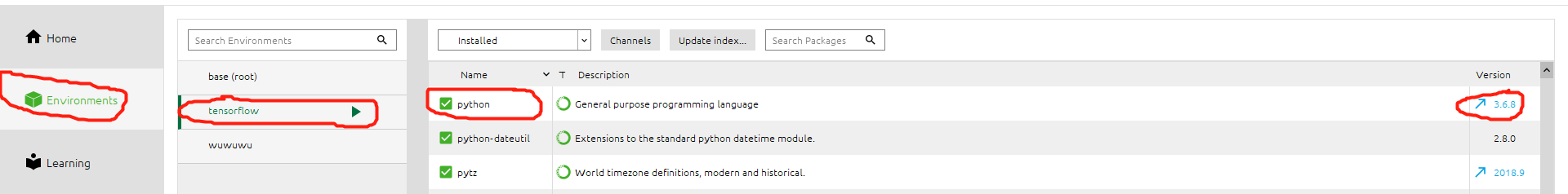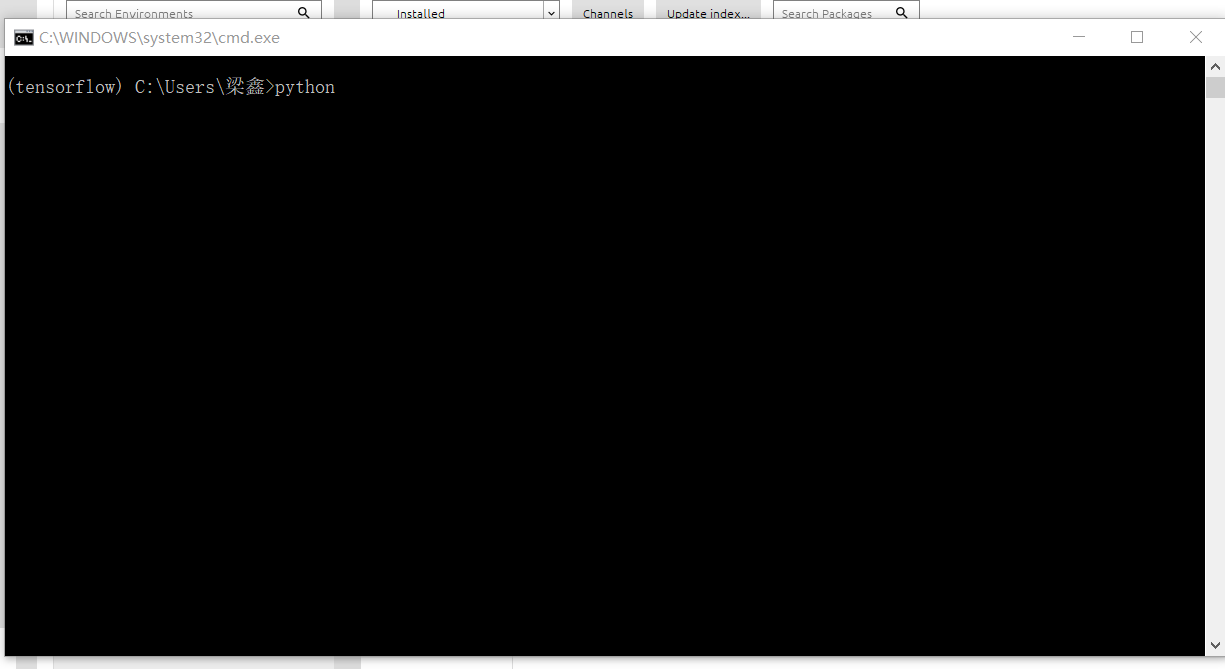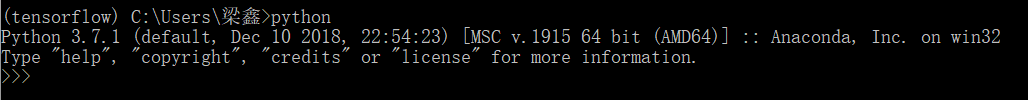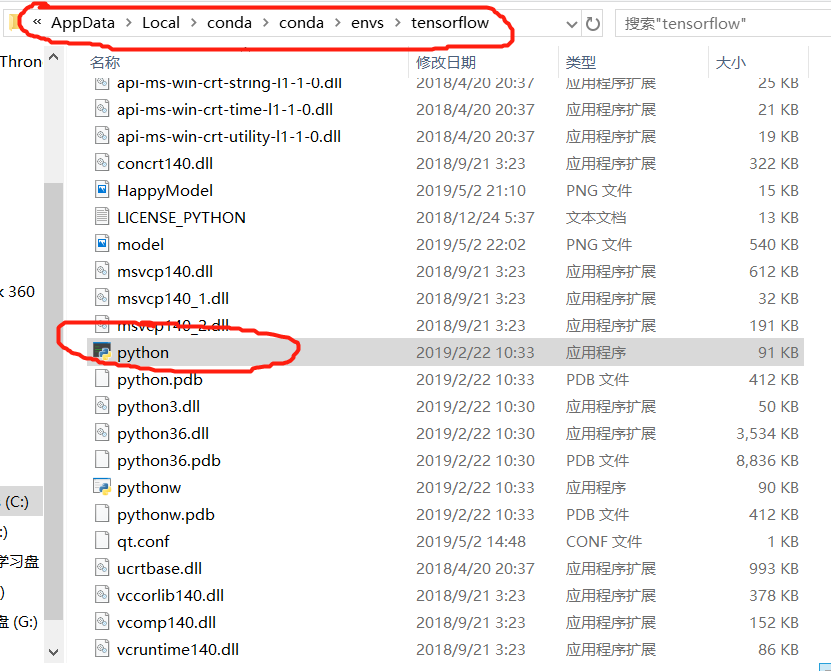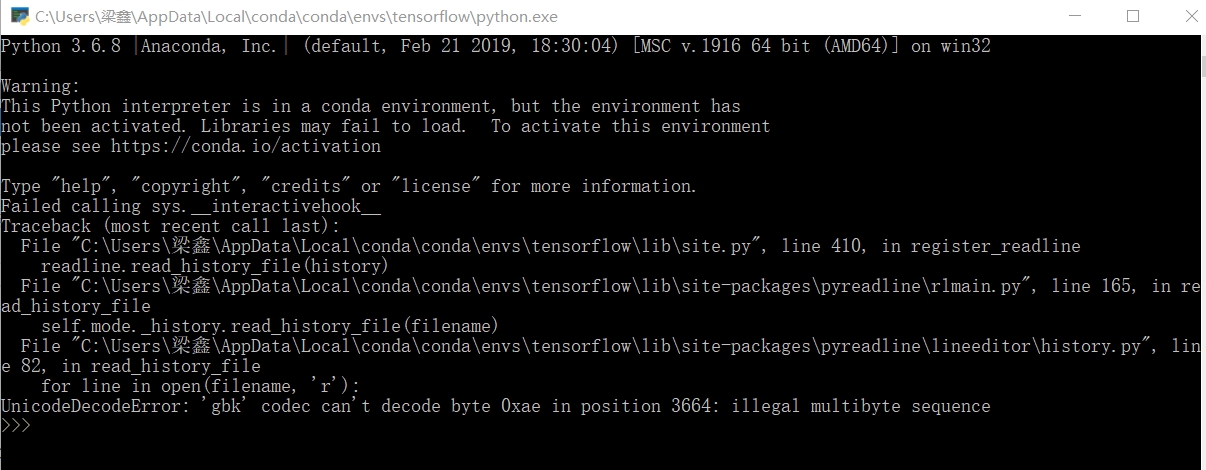Anaconda激活python3的环境后在终端中使用的python版本确实python2(Mac环境)

theano 报错 module 'configparser' has no attribute 'ConfigParser' 用的是Anaconda3 python3.6

>theano 报错 module 'configparser' has no attribute 'ConfigParser' 用的是Win10 Anaconda3 python3.6  from sklearn.datasets import load_boston import theano.tensor as T import numpy as np import matplotlib.pyplot as plt import theano class Layer(object): def __init__(self,inputs,in_size,out_size,activation_function=None): self.W = theano.shared(np.random.normal(0,1,(in_size,out_size))) self.b = theano.shared(np.zeros((out_size,)) + 0.1) self.Wx_plus_b = T.dot(inputs, self.W) + self.b self.activation_function = activation_function if activation_function is None: self.outputs = self.Wx_plus_b else: self.outputs = self.activation_function(self.Wx_plus_b) def minmax_normalization(data): xs_max = np.max(data, axis=0) xs_min = np.min(data, axis=0) xs = (1-0)*(data - xs_min)/(xs_max - xs_min) + 0 return xs np.random.seed(100) x_dataset = load_boston() x_data = x_dataset.data # minmax normalization, rescale the inputs x_data = minmax_normalization(x_data) y_data = x_dataset.target[:,np.newaxis] #cross validation, train test data split x_train, y_train = x_data[:400], y_data[:400] x_test, y_test = x_data[400:], y_data[400:] x = T.dmatrix('x') y = T.dmatrix('y') l1 = Layer(x, 13, 50, T.tanh) l2 = Layer(l1.outputs, 50, 1, None) #compute cost cost = T.mean(T.square(l2.outputs - y)) #cost = T.mean(T.square(l2.outputs - y)) + 0.1*((l1.W**2).sum() + (l2.W**2).sum()) #l2 regulization #cost = T.mean(T.square(l2.outputs - y)) + 0.1*(abs(l1.W).sum() + abs(l2.W).sum()) #l1 regulization gW1, gb1, gW2, gb2 = T.grad(cost, [l1.W,l1.b,l2.W,l2.b]) #gradient descend learning_rate = 0.01 train = theano.function(inputs=[x,y], updates=[(l1.W,l1.W-learning_rate*gW1), (l1.b,l1.b-learning_rate*gb1), (l2.W,l2.W-learning_rate*gW2), (l2.b,l2.b-learning_rate*gb2)]) compute_cost = theano.function(inputs=[x,y], outputs=cost) #record cost train_err_list = [] test_err_list = [] learning_time = [] for i in range(1000): if 1%10 == 0: #record cost train_err_list.append(compute_cost(x_train,y_train)) test_err_list.append(compute_cost(x_test,y_test)) learning_time.append(i) #plot cost history plt.plot(learning_time, train_err_list, 'r-') plt.plot(learning_time, test_err_list,'b--') plt.show() #作者 morvan莫凡 https://morvanzhou.github.io  报错了： Traceback (most recent call last): File "C:/Users/Elena/PycharmProjects/theano/regularization.py", line 1, in <module> from sklearn.datasets import load_boston File "C:\Users\Elena\Anaconda3\lib\site-packages\sklearn\datasets\__init__.py", line 22, in <module> from .twenty_newsgroups import fetch_20newsgroups File "C:\Users\Elena\Anaconda3\lib\site-packages\sklearn\datasets\twenty_newsgroups.py", line 44, in <module> from ..feature_extraction.text import CountVectorizer File "C:\Users\Elena\Anaconda3\lib\site-packages\sklearn\feature_extraction\__init__.py", line 10, in <module> from . import text File "C:\Users\Elena\Anaconda3\lib\site-packages\sklearn\feature_extraction\text.py", line 28, in <module> from ..preprocessing import normalize File "C:\Users\Elena\Anaconda3\lib\site-packages\sklearn\preprocessing\__init__.py", line 6, in <module> from ._function_transformer import FunctionTransformer File "C:\Users\Elena\Anaconda3\lib\site-packages\sklearn\preprocessing\_function_transformer.py", line 5, in <module> from ..utils.testing import assert_allclose_dense_sparse File "C:\Users\Elena\Anaconda3\lib\site-packages\sklearn\utils\testing.py", line 61, in <module> from nose.tools import raises as _nose_raises File "C:\Users\Elena\Anaconda3\lib\site-packages\nose\__init__.py", line 1, in <module> from nose.core import collector, main, run, run_exit, runmodule File "C:\Users\Elena\Anaconda3\lib\site-packages\nose\core.py", line 11, in <module> from nose.config import Config, all_config_files File "C:\Users\Elena\Anaconda3\lib\site-packages\nose\config.py", line 6, in <module> import configparser File "C:\Users\Elena\Anaconda3\Lib\site-packages\theano\configparser.py", line 15, in <module> import theano File "C:\Users\Elena\Anaconda3\lib\site-packages\theano\__init__.py", line 88, in <module> from theano.configdefaults import config File "C:\Users\Elena\Anaconda3\lib\site-packages\theano\configdefaults.py", line 17, in <module> from theano.configparser import (AddConfigVar, BoolParam, ConfigParam, EnumStr, File "C:\Users\Elena\Anaconda3\lib\site-packages\theano\configparser.py", line 77, in <module> theano_cfg = (configparser.ConfigParser if PY3 **AttributeError: module 'configparser' has no attribute 'ConfigParser**' 把theano里的configparser.py文件里的ConfigParser改成了configparser还是不行 换了模块import configparsor也不行。。。![图片说明](https://img-ask.csdn.net/upload/201909/30/1569832318_223436.png)

anaconda创建新环境后python版本的问题

linux anaconda3 python环境，import dlib报错

Mac下的Anaconda 无法使用matplotlib.pyplot

File "/anaconda3/lib/python3.6/site-packages/matplotlib/__init__.py", line 891, in __getitem__ from matplotlib import pyplot as plt File "/anaconda3/lib/python3.6/site-packages/matplotlib/pyplot.py", line 32, in <module> import matplotlib.colorbar File "/anaconda3/lib/python3.6/site-packages/matplotlib/colorbar.py", line 28, in <module> import matplotlib.artist as martist AttributeError: module 'matplotlib' has no attribute 'artist'

python3.6 anaconda3，prompt显示“此时不应有...”

Anaconda3.6的pyodbc无法import

windows下装了Anaconda 3.6，我看到官方文档和conda list的结果都是自带pyodbc 4.0.16的，但是在eclipse中import时找不到，后来到site-packages里看，只有pyodbc的pyd和egg-info文件，没有module文件夹。请问为什么会这样，怎么解决？谢谢。

Pycharm解释器指向anaconda的python怎么装package

ArrayList源码分析(入门篇)

ArrayList源码分析 前言： 写这篇博客的主要原因是，在我上一次参加千牵科技Java实习生面试时，有被面试官问到ArrayList为什么查找的速度较快，插入和删除的速度较慢？当时我回答得不好，很大的一部分原因是因为我没有阅读过ArrayList源码，虽然最后收到Offer了，但我拒绝了，打算寒假学得再深入些再广泛些，下学期开学后再去投递其他更好的公司。为了更加深入理解ArrayList，也为

String s = new String(" a ") 到底产生几个对象？

loonggg读完需要3分钟速读仅需 1 分钟大家好，我是你们的校长。我之前讲过，这年头，只要肯动脑，肯行动，程序员凭借自己的技术，赚钱的方式还是有很多种的。仅仅靠在公司出卖自己的劳动时...

MySQL数据库面试题（2020最新版）

HashMap底层实现原理，红黑树，B+树，B树的结构原理 Spring的AOP和IOC是什么？它们常见的使用场景有哪些？Spring事务，事务的属性，传播行为，数据库隔离级别 Spring和SpringMVC，MyBatis以及SpringBoot的注解分别有哪些？SpringMVC的工作原理，SpringBoot框架的优点，MyBatis框架的优点 SpringCould组件有哪些，他们...

《经典算法案例》01-08：如何使用质数设计扫雷（Minesweeper）游戏